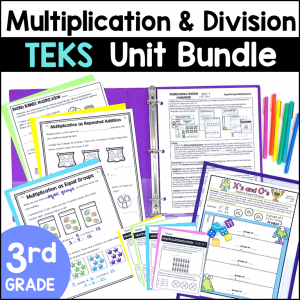Save 10% on your first order with code EXTRA10

# 3rd Grade Multiplication and Division Worksheets – TEKS

\$4.00\$29.99
SKU: 8592407 Category:

## Description

These No Prep Worksheets are perfect to practice Multiplication and Division skills! There are fun & engaging pages teach multiplication and division word problems and concepts. Students get fun practice on arrays, jumps on a number line, relationships between multiplication and division, and much more!

What’s Included:

• 31 student pages
• Complete Teacher Key

Great ways to use these Worksheets:

• Seatwork
• Math Stations
• Tutoring
• Review
• Small Group Reteach

Topics include:

• One-step Multiplication and Division Word Problems
• Arrays
• Representing Multiplication with Repeated Addition, Skip Counting, Jumps on a Number Line, Area Models and Arrays
• Commutative Property of Multiplication
• Distributive Property of Multiplication
• Understanding the Multiplication Chart
• Relationships between Multiplication and Division
• Division of objects into Equal Groups or taking out Equal Sets
• Representing Division with Repeated Subtraction, Skip Counting, Backwards Jumps on a Number Line, and Arrays
• Even and Odd Numbers
• Describing Multiplication as a Comparison
• Finding Missing Numbers in Multiplication and Division equations

TEKS Alignment:

Supporting Standards

3.4D: Determine the total number of objects when equally sized groups of objects are combined or arranged in arrays up to 10 by 10.

3.4E: Represent multiplication facts by using a variety of approaches such as repeated addition, equal-sized groups, arrays, area models, equal jumps on a number line, and skip counting.

3.4F: Recall facts to multiply up to 10 by 10 with automaticity and recall the corresponding division facts.

3.4H: Determine the number of objects in each group when a set of objects is partitioned into equal shares or a set of objects is shared equally.

3.4I: Determine if a number is even or odd using divisibility rules.

3.4J: Determine a quotient using the relationship between multiplication and division.

3.5C: Describe a multiplication expression as a comparison such as 3 x 24 represents 3 times as much as 24.

3.5D: Determine the unknown whole number in a multiplication or division equation relating three whole numbers when the unknown is either a missing factor or product.